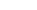•WE PROVIDEPRE CALCULUS
HOMEWORK
HELP
We can help with
Systems of Equations
Geometry
Trigonometry
and much more
starts at \$25/hour.

# Pre Calculus Homework Help

## Pre Calculus Topics

• Algebra
• Systems of Equations.
• Word problems.
• Factorization.
• Polynomials

• Geometry
• Triangles.
• Areas and Volumes.
• Circles.
• Lines
• Planes

• Trigonometry
• Circles.
• Sine, Cosine, Tangent, Cotangent, Secant, Cosecant.
• Pythagoras Theorem.
• De Moivre's Formula.
• etc....

## Claim Your Free Solved Stats Problems!

* indicates required
Email Format

## Our Specialties

#### Algebra

There are many Algebra topics covered in a Pre Calculus class, such as Systems of Equations, Word problems, Factorization, Polynomials, Quadratic Equations.

#### Geometry

We can help you with your Geometry homework, involving Triangles, Areas and Volumes, Circles, Lines, Planes, etc.

#### Trigonometry

Are you stuck with a Trigonometry assignment? Are you lost with Circles or with trigonometric functions such as Sine, Cosine, Tangent, Cotangent, Secant and Co-secant? We can help with that!

#### Basic Calculus

You will need to have some idea of Calculus to work on your pre-calculus course. We can cover you!

#### We provide help with ALL Math Subjects

Aside from Pre Calculus, we can also help with Stats, Calculus, Linear Algebra, Quantitative Methods, Decision Analysis, you name it

#### We are a partner you can trust

We have been online more than 10 years and we have worked successfully with thousands of satisfied customers

$3x+2y=11 \,\,\,\,\,\,\,\,\,(1)$ $5x-4y=11 \,\,\,\,\,\,\,\,\, (2)$ $6x+4y=22 \,\,\,\,\,\,\,\,\,(3)$ $5x-4y=11 \,\,\,\,\,\,\,\,\, (4)$ $x=3 \quad , \quad y=1$ $\displaystyle{ \frac{\sqrt{2}}{\sqrt 3-\sqrt 2}}$ $\displaystyle \frac{\sqrt{2}}{\sqrt 3-\sqrt 2}= \frac{\sqrt{2} (\sqrt 3+\sqrt 2)}{(\sqrt 3-\sqrt 2)(\sqrt 3+\sqrt 2)} = \frac{\sqrt 2 \sqrt 3 + (\sqrt 2)^2}{(\sqrt 3)^2-(\sqrt 2)^2}$ $\displaystyle = \frac{\sqrt 2 \sqrt 3 + (\sqrt 2)^2}{3-2}=\sqrt 6-2$ $\displaystyle \frac{\sqrt{2}}{\sqrt 3-\sqrt 2}=\sqrt 6-2$